# NCERT solutions for Mathematics Exemplar Class 9 chapter 3 - Coordinate Geometry [Latest edition]

#### Chapters## Chapter 3: Coordinate Geometry

Exercise 3.1Exercise 3.2Exercise 3.3Exercise 3.4
Exercise 3.1 [Pages 25 - 27]

### NCERT solutions for Mathematics Exemplar Class 9 Chapter 3 Coordinate Geometry Exercise 3.1 [Pages 25 - 27]

#### Choose the correct alternative:

Exercise 3.1 | Q 1 | Page 25

Point (–3, 5) lies in the ______.

Exercise 3.1 | Q 2 | Page 25

Signs of the abscissa and ordinate of a point in the second quadrant are respectively ______.

• +, +

• –, –

• –, +

• +, –

Exercise 3.1 | Q 3 | Page 25

Point (0, –7) lies ______.

• On the x –axis

• On the y-axis

Exercise 3.1 | Q 4 | Page 25

Point (– 10, 0) lies ______.

• On the negative direction of the x-axis

• On the negative direction of the y-axis

Exercise 3.1 | Q 5 | Page 25

Abscissa of all the points on the x-axis is ______.

• 0

• 1

• 2

• Any number

Exercise 3.1 | Q 6 | Page 25

Ordinate of all points on the x-axis is ______.

• 0

• 1

• – 1

• Any number

Exercise 3.1 | Q 7 | Page 26

The point at which the two coordinate axes meet is called the ______.

• Abscissa

• Ordinate

• Origin

Exercise 3.1 | Q 8 | Page 26

A point both of whose coordinates are negative will lie in ______.

Exercise 3.1 | Q 9 | Page 26

Points (1, – 1), (2, – 2), (4, – 5), (– 3, – 4) ______.

• Do not lie in the same quadrant

Exercise 3.1 | Q 10 | Page 26

If y-coordinate of a point is zero, then this point always lies ______.

• On x-axis

• On y-axis

Exercise 3.1 | Q 11 | Page 26

The points (– 5, 2) and (2, – 5) lie in the ______.

• II and III quadrants, respectively

• II and IV quadrants, respectively

• IV and II quadrants, respectively

Exercise 3.1 | Q 12 | Page 26

If the perpendicular distance of a point P from the x-axis is 5 units and the foot of the perpendicular lies on the negative direction of x-axis, then the point P has ______.

• x-coordinate = – 5

• y-coordinate = 5 only

• y-coordinate = – 5 only

• y-coordinate = 5 or –5

Exercise 3.1 | Q 13 | Page 26

On plotting the points O(0, 0), A(3, −4), B(3, 4) and C(0, 4) and joining OA, AB, BC and CO, which of the following figure is obtained?

• Square

• Rectangle

• Trapezium

• Rhombus

Exercise 3.1 | Q 14 | Page 26

If P(– 1, 1), Q(3, – 4), R(1, –1), S(–2, –3) and T(– 4, 4) are plotted on the graph paper, then the point(s) in the fourth quadrant are ______.

• P and T

• Q and R

• Only S

• P and R

Exercise 3.1 | Q 15 | Page 26

If the coordinates of the two points are P(–2, 3) and Q(–3, 5), then (abscissa of P) – (abscissa of Q) is ______.

• – 5

• 1

• – 1

• – 2

Exercise 3.1 | Q 16 | Page 26

If P(5, 1), Q(8, 0), R(0, 4), S(0, 5) and O(0, 0) are plotted on the graph paper, then the point(s) on the x-axis are ______.

• P and R

• R and S

• Only Q

• Q and O

Exercise 3.1 | Q 17 | Page 26

Abscissa of a point is positive in ______.

Exercise 3.1 | Q 18 | Page 27

The points whose abscissa and ordinate have different signs will lie in ______.

Exercise 3.1 | Q 19 | Page 27

In figure, coordinates of P are ______.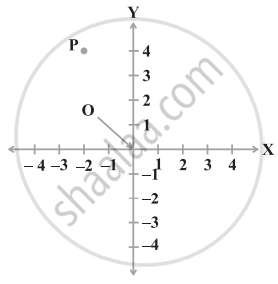• (– 4, 2)

• (– 2, 4)

• (4, – 2)

• (2, – 4)

Exercise 3.1 | Q 20 | Page 27

In figure, the point identified by the coordinates (–5, 3) is ______.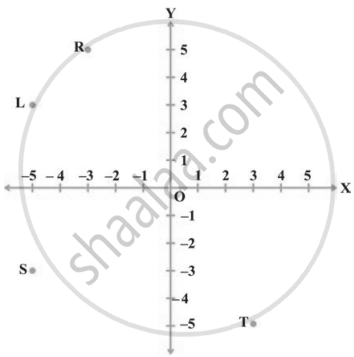• T

• R

• L

• S

Exercise 3.1 | Q 21 | Page 27

The point whose ordinate is 4 and which lies on y-axis is ______.

• (4, 0)

• (0, 4)

• (1, 4)

• (4, 2)

Exercise 3.1 | Q 22 | Page 27

Which of the points P(0, 3), Q(1, 0), R(0, – 1), S(–5, 0), T(1, 2) do not lie on the x-axis?

• P and R only

• Q and S only

• P, R and T

• Q, S and T

Exercise 3.1 | Q 23 | Page 27

The point which lies on y-axis at a distance of 5 units in the negative direction of y-axis is ______.

• (0, 5)

• (5, 0)

• (0, – 5)

• (– 5, 0)

Exercise 3.1 | Q 24 | Page 27

The perpendicular distance of the point P (3, 4) from the y-axis is ______.

• 3

• 4

• 5

• 7

Exercise 3.2 [Page 28]

### NCERT solutions for Mathematics Exemplar Class 9 Chapter 3 Coordinate Geometry Exercise 3.2 [Page 28]

#### State whether the statement is True or False

Exercise 3.2 | Q 1.(i) | Page 28

Point (3, 0) lies in the first quadrant.

• True

• False

Exercise 3.2 | Q 1.(ii) | Page 28

Points (1, –1) and (–1, 1) lie in the same quadrant.

• True

• False

Exercise 3.2 | Q 1.(iii) | Page 28

The coordinates of a point whose ordinate is -1/2 and abscissa is 1 are -1/2, 1.

• True

• False

Exercise 3.2 | Q 1.(iv) | Page 28

A point lies on y-axis at a distance of 2 units from the x-axis. Its coordinates are (2, 0).

• True

• False

Exercise 3.2 | Q 1.(v) | Page 28

(–1, 7) is a point in the II quadrant

• True

• False

Exercise 3.3 [Pages 29 - 31]

### NCERT solutions for Mathematics Exemplar Class 9 Chapter 3 Coordinate Geometry Exercise 3.3 [Pages 29 - 31]

Exercise 3.3 | Q 1 | Page 29

Write the coordinates of each of the points P, Q, R, S, T and O from the figure.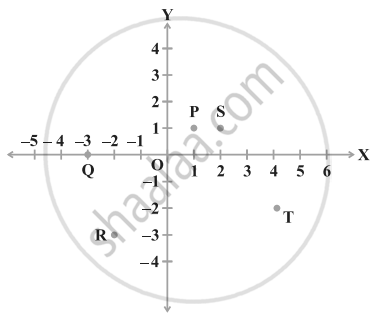Exercise 3.3 | Q 2 | Page 30

Plot the following points and write the name of the figure obtained by joining them in order: P(– 3, 2), Q(– 7, – 3), R(6, – 3), S(2, 2)

Exercise 3.3 | Q 3 | Page 30

Plot the points (x, y) given by the following table:

 x 2 4 – 3 – 2 3 0 y 4 2 0 5 – 3 0
Exercise 3.3 | Q 4.(i) | Page 30

Plot the following points and check whether they are collinear or not:

(1, 3), (– 1, – 1), (– 2, – 3)

Exercise 3.3 | Q 4.(ii) | Page 30

Plot the following points and check whether they are collinear or not:

(1, 1), (2, – 3), (– 1, – 2)

Exercise 3.3 | Q 4.(iii) | Page 30

Plot the following points and check whether they are collinear or not:

(0, 0), (2, 2), (5, 5)

Exercise 3.3 | Q 5.(i) | Page 30

Without plotting the points indicate the quadrant in which they will lie, if ordinate is 5 and abscissa is – 3

Exercise 3.3 | Q 5.(ii) | Page 30

Without plotting the points indicate the quadrant in which they will lie, if abscissa is – 5 and ordinate is – 3

Exercise 3.3 | Q 5.(iii) | Page 30

Without plotting the points indicate the quadrant in which they will lie, if abscissa is – 5 and ordinate is 3

Exercise 3.3 | Q 5.(iv) | Page 30

Without plotting the points indicate the quadrant in which they will lie, if ordinate is 5 and abscissa is 3

Exercise 3.3 | Q 6.(i) | Page 30

In figure, LM is a line parallel to the y-axis at a distance of 3 units. What are the coordinates of the points P, R and Q?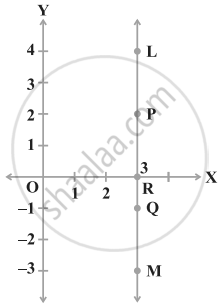Exercise 3.3 | Q 6.(ii) | Page 30

In figure, LM is a line parallel to the y-axis at a distance of 3 units. What is the difference between the abscissa of the points L and M?Exercise 3.3 | Q 7.(i) | Page 30

In which quadrant or on which axis the following points lie?

(– 3, 5),

Exercise 3.3 | Q 7.(ii) | Page 30

In which quadrant or on which axis the following points lie?

(4, – 1)

Exercise 3.3 | Q 7.(iii) | Page 30

In which quadrant or on which axis the following points lie?

(2, 0)

Exercise 3.3 | Q 7.(iv) | Page 30

In which quadrant or on which axis the following points lie?

(2, 2)

Exercise 3.3 | Q 7.(v) | Page 30

In which quadrant or on which axis the following points lie?

(– 3, – 6)

Exercise 3.3 | Q 8 | Page 30

Which of the following points lie on y-axis?

A(1, 1), B(1, 0), C(0, 1), D(0, 0), E(0, – 1), F(– 1, 0), G(0, 5), H(– 7, 0), I(3, 3).

Exercise 3.3 | Q 9 | Page 30

Plot the points (x, y) given by the following table. Use scale 1 cm = 0.25 units

 x 1.25 0.25 1.5 – 1.75 y – 0.5 1 1.5 – 0.25
Exercise 3.3 | Q 10 | Page 31

A point lies on the x-axis at a distance of 7 units from the y-axis. What are its coordinates? What will be the coordinates if it lies on y-axis at a distance of –7 units from x-axis?

Exercise 3.3 | Q 11.(i) | Page 31

Find the coordinates of the point which lies on x and y axes both.

Exercise 3.3 | Q 11.(ii) | Page 31

Find the coordinates of the point whose ordinate is – 4 and which lies on y-axis.

Exercise 3.3 | Q 11.(iii) | Page 31

Find the coordinates of the point whose abscissa is 5 and which lies on x-axis.

Exercise 3.3 | Q 12 | Page 31

Taking 0.5 cm as 1 unit, plot the following points on the graph paper: A(1, 3), B(– 3, – 1), C(1, – 4), D(– 2, 3), E(0, – 8), F(1, 0)

Exercise 3.4 [Page 32]

### NCERT solutions for Mathematics Exemplar Class 9 Chapter 3 Coordinate Geometry Exercise 3.4 [Page 32]

Exercise 3.4 | Q 1 | Page 32

Points A(5, 3), B(– 2, 3) and D(5, – 4) are three vertices of a square ABCD. Plot these points on a graph paper and hence find the coordinates of the vertex C.

Exercise 3.4 | Q 2 | Page 32

Write the coordinates of the vertices of a rectangle whose length and breadth are 5 and 3 units respectively, one vertex at the origin, the longer side lies on the x-axis and one of the vertices lies in the third quadrant.

Exercise 3.4 | Q 3 | Page 32

Plot the points P(1, 0), Q(4, 0) and S(1, 3). Find the coordinates of the point R such that PQRS is a square.

Exercise 3.4 | Q 4.(i) | Page 32

From the figure, answer the following:

Write the points whose abscissa is 0.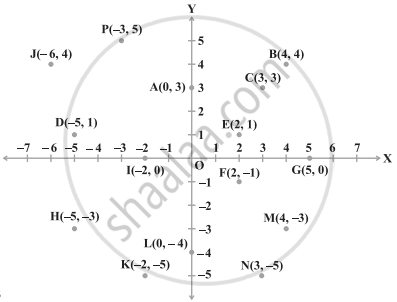Exercise 3.4 | Q 4.(ii) | Page 32

From the figure, answer the following:

Write the points whose ordinate is 0.Exercise 3.4 | Q 4.(iii) | Page 32

From the figure, answer the following:

Write the points whose abscissa is – 5.Exercise 3.4 | Q 5.(i) | Page 32

Plot the points A(1, – 1) and B(4, 5). Draw a line segment joining these points. Write the coordinates of a point on this line segment between the points A and B.

Exercise 3.4 | Q 5.(ii) | Page 32

Plot the points A(1, – 1) and B(4, 5). Extend this line segment and write the coordinates of a point on this line which lies outside the line segment AB.

## Chapter 3: Coordinate Geometry

Exercise 3.1Exercise 3.2Exercise 3.3Exercise 3.4## NCERT solutions for Mathematics Exemplar Class 9 chapter 3 - Coordinate Geometry

NCERT solutions for Mathematics Exemplar Class 9 chapter 3 (Coordinate Geometry) include all questions with solution and detail explanation. This will clear students doubts about any question and improve application skills while preparing for board exams. The detailed, step-by-step solutions will help you understand the concepts better and clear your confusions, if any. Shaalaa.com has the CBSE Mathematics Exemplar Class 9 solutions in a manner that help students grasp basic concepts better and faster.

Further, we at Shaalaa.com provide such solutions so that students can prepare for written exams. NCERT textbook solutions can be a core help for self-study and acts as a perfect self-help guidance for students.

Concepts covered in Mathematics Exemplar Class 9 chapter 3 Coordinate Geometry are Cartesian Coordinate System, Plotting a Point in the Plane If Its Coordinates Are Given., Coordinate Geometry.

Using NCERT Class 9 solutions Coordinate Geometry exercise by students are an easy way to prepare for the exams, as they involve solutions arranged chapter-wise also page wise. The questions involved in NCERT Solutions are important questions that can be asked in the final exam. Maximum students of CBSE Class 9 prefer NCERT Textbook Solutions to score more in exam.

Get the free view of chapter 3 Coordinate Geometry Class 9 extra questions for Mathematics Exemplar Class 9 and can use Shaalaa.com to keep it handy for your exam preparation